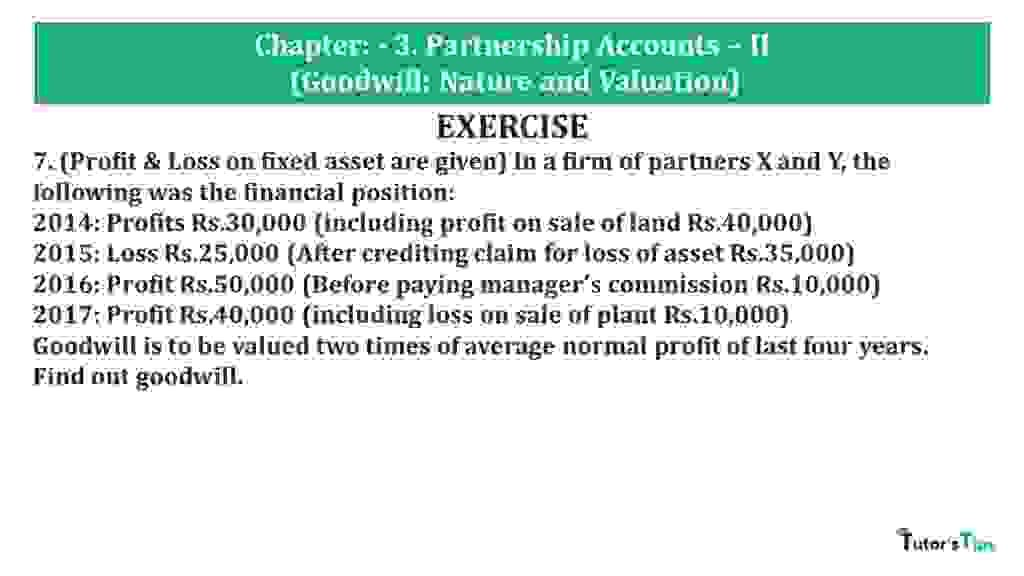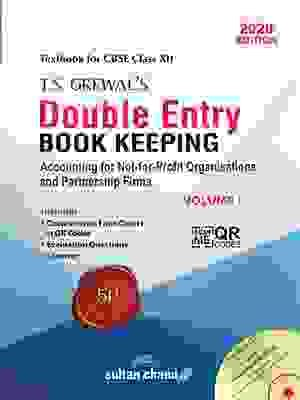# Question 07 Chapter 3 of +2 Part-1 – USHA Publication 12 Class Part – 1Question 07 Chapter 3 of +2-Part-1

7. (Profit & Loss on fixed asset are given) In a firm of partners X and Y, the following was the financial position:
2014: Profits Rs.30,000 (including profit on sale of land Rs.40,000)
2015: Loss Rs.25,000 (After crediting claim for loss of asset Rs.35,000)
2016: Profit Rs.50,000 (Before paying manager’s commission Rs.10,000)
2017: Profit Rs.40,000 (including loss on sale of plant Rs.10,000)
Goodwill is to be valued two times of average normal profit of last four years. Find out goodwill.

### The solution of Question 07 Chapter 3 of +2 Part-1: –

 Average Profit = Total Profit for past given years Number of years = (-10,000) + (-60,000) + 40,000 + 50,000 4 = 20,000 4 = 5,000

 Number of years’ purchase = 2 Goodwill = Average Profit X Number of years’ purchase Goodwill = 5,000 X 2 Goodwill = 10,000

Working Note : –

*1 Calculation of Profits of last three years

 Adjusted Profit for 1st year = Total Profit −Interest on capital = 30,000 – 40,000 = (-10,000)

 Adjusted Profit for 2nd year = Total Profit – Claim for loss of asset = -(25,000) – 35,000 = (-60,000)

 Adjusted Profit for 3rd year = Total Profit − Interest on capital = 50,000 – 10,000 = 40,000

 Adjusted Profit for 3rd year = Total Profit − Interest on capital = 40,000 + 10,000 = 50,000

Thanks, Please Like and share with your friends

Comment if you have any questions.

Also, Check out the solved question of previous Chapters: –

## Usha Publication – Accountancy PSEB (Class 12) – Volume II – SolutionVol. I: Accounting for Not-for-Profit Organizations and Partnership Firms